Filter search results
Sort by
Content type
Image orientation
Color composition
People
Number of people
Gender
Age group
Ethnicity
More filters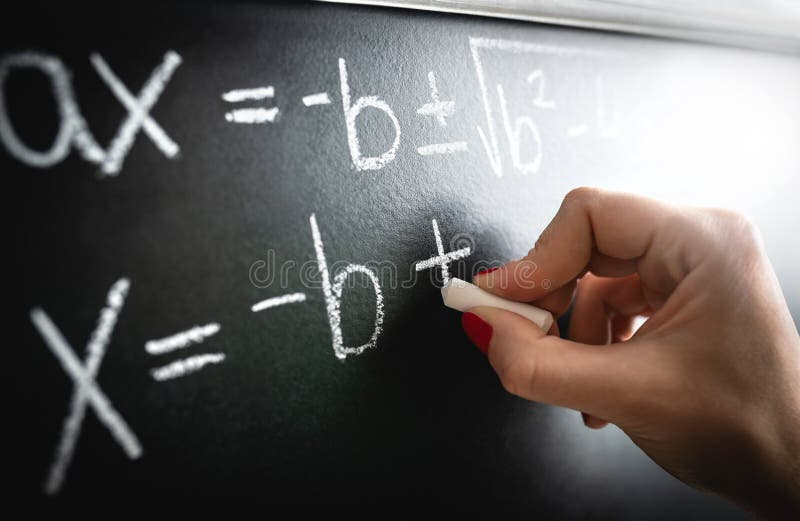Math equation, function or calculation on chalkboard. Teacher writing on blackboard during lesson and lecture in school. Math equation, function or calculation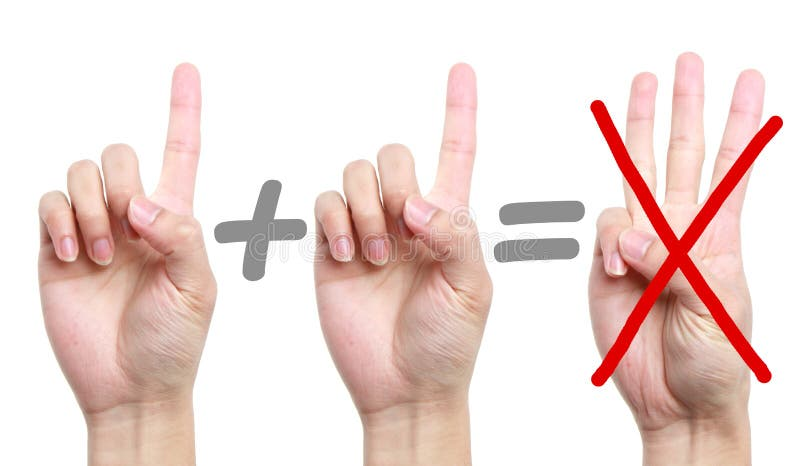Error Calculation. Fingers showing error calculation one plus one equal threeConcept of taxes paid by individuals and corporations such as vat, income and wealth tax. Tax payment. State taxes. Calculation. Tax returnTwo piles of boxes on the scales. Trade balance and calculation by barter. Bypassing sanctions, import and export of goods. Trade. Balance. Goods turnoverStrain face Asian woman hand holding expense bill and calculation about debt bills monthly at the table in home office. Strain face Asian woman hand holding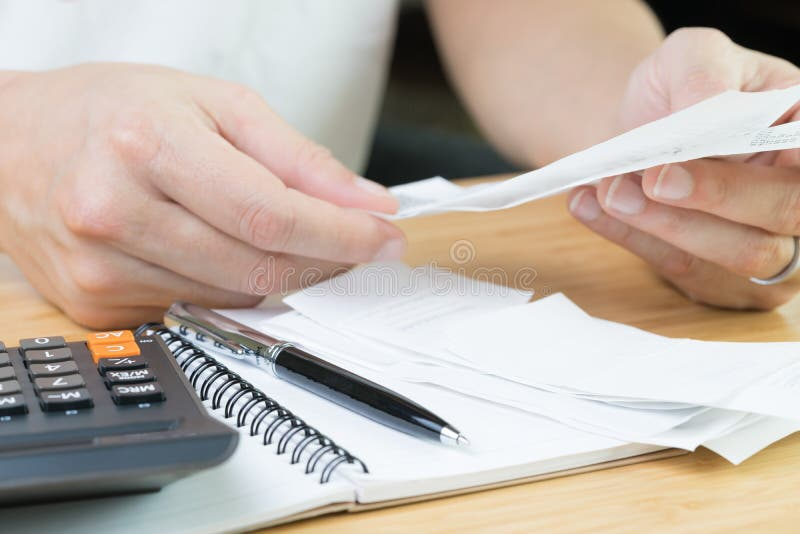Accounting, cost or profit and loss calculation concept, hand holding financial expense bills or receipt with calculator and pen. On small notebook on woodenSenior man doing calculation at home. Senior man busy doing calculation, counting money and bills at home, sitting at deskBusinessman working doing finances and calculation cost of real estate investment while be signing to contract, Concept mortgage. Loan approval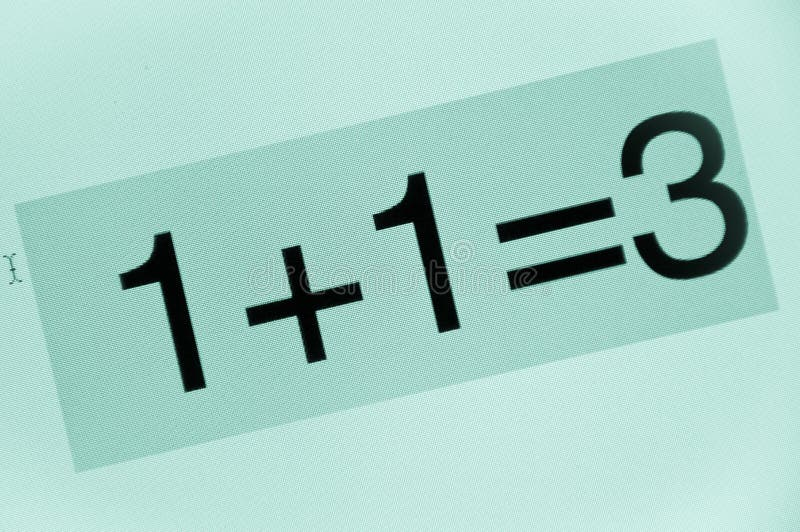Bad calculation. Close up image of computer screen with bad calculation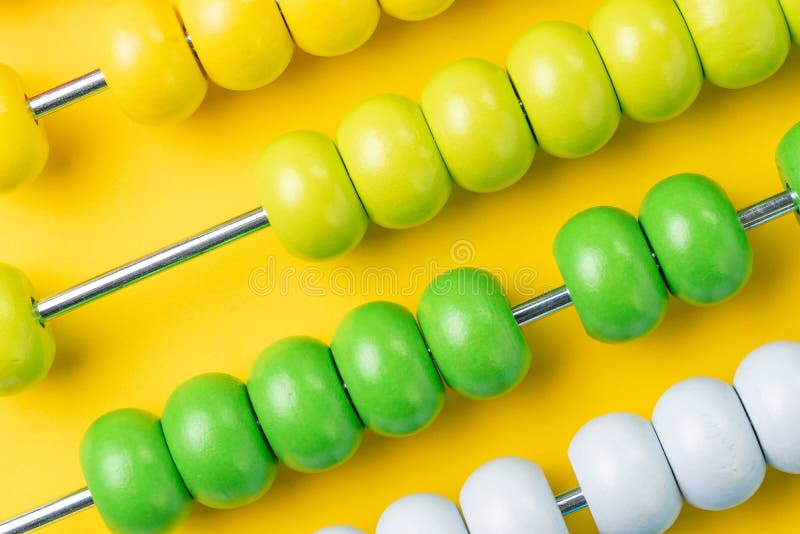Colorful wooden abacus beads on yellow background, business financial or accounting cost and expense calculation concept, or use. In education school arithmeticCalculation sheet. With business graphCalculation and put an order. A ball pen and a calculator putting on order sheet, shown as successful selling or business purchase, or an order after carefulCalculation account. Financial chart news on a newspaper page. business account. calculationHome key with house keyring or keychain with white calculator on wooden table using as mortgage calculation or buy and sell. Property and real estateClose up costs in the calculation of business on printing receipts at her desk with a calculator.Tax Self Assesment and Accounting Calculation. Tax Return Self Assesment Form and CalculationMath teacher writing function, equation or calculation on blackboard in school classroom. Student calculating on chalkboard. Substitute professor workingTax Self Assesment and Accounting Calculation. Tax Return Self Assesment Form and CalculationCalculation. Tools on white backgroundTax calculation. Concept shot of tax calculationCalculator for calculating the numbers accounting accountancy business calculation on white background side viewHand man pressing on calculator with calculation about tax annual at home office.Hard mathematics calculation. Rear view of a puzzled businessman with a lightbulb sketch above head having project ideas and business planning concept isolatedCost benefit calculation analysis finances in company business c. Cost benefit calculation analysis expenses finances in company business conceptCalculation of the utility bills. Scene with pen, bills, calculator and coffeeSenior hispanic man busy doing calculation, counting money and bills at home. He is looking through his taxesAssessment Evaluation Opinion Analysis Calculation Concept.Calculation of the utility bills. Scene with pen, bills, calculator and coffeeAssessment Calculation Estimate Evaluate Measurement ConceptSad Asian woman hand holding expense bill and calculation about debt bills monthly at the table in home office. Sad Asian woman hand holding expense bill andTax calculation. Concept shot of tax calculationMagnifying glass looks at money bag with coins and a calculator. Profit calculation and income analysis. Interest rates. Profitability. Performance. MoneyThe man is sitting thinking over the sorted money. Expense planning and financial issues. Calculation of property tax, payment. Of utilities. Counting the costsInitial coin offering ICO with coins, calculation table, office tools on gray textured background top view. Crypto currency. Initial coin offering ICO with coinsLogarithmic calculation table book, macro photo. Close up of logarithmic calculation table, printed in an old bookWoman doing financial calculation. Attractive young woman doing financial calculation at home, using calculator, counting moneyBusinessperson Doing Calculation. Close-up Of Businessperson Hands Doing Calculation At DeskWorker in a black hat, records the calculation of further work on the tabletA worker in a black hat measures a wooden balcony with a meter and records the calculation on a tabletCarpenter making project calculation using smartphone app. In small business woodwork workshopBusinessperson doing calculation. Close-up Of Businessperson Doing Calculation On Calculator With DocumentStem education concept. study algebra at university. back to school. teacher of arithmetic. calculation and search of. Various data. precision measurement toolStudy hard. Back to school. Teacher of stem. Calculation and data. Measurement tool. Measuring angle. School education. Free education. Teaching you. BeardedStem education concept. study algebra at university. back to school. teacher of arithmetic. calculation and search of. Various data. precision measurement toolCalculation and search of various data. precision measurement tool. man use triangle. math or mathematics. Stem. Education concept. study algebra at universityCalculation and search of various data. precision measurement tool. man use triangle. math or mathematics. Stem. Education concept. study algebra at universityStem education concept. study algebra at university. back to school. teacher of arithmetic. calculation and search of. Various data. precision measurement toolTeaching occupation vacant job. Bearded man use protractor. Education concept. Study hard. Teacher of stem. Calculation. And data. Measurement tool. MeasuringBack to school. teacher of arithmetic. calculation and search of various data. precision measurement tool. man use. Triangle. math or mathematics. StemStudy algebra at university. back to school. teacher of arithmetic. calculation and search of various data. precision. Measurement tool. man use triangle. mathTeacher of arithmetic. calculation and search of various data. precision measurement tool. man use triangle. math or. Mathematics. Stem education concept. studyTeacher of arithmetic. calculation and search of various data. precision measurement tool. man use triangle. math or. Mathematics. Stem education concept. studyYoung student getting crazy with maths calculation. Young Asian student getting crazy with maths calculation studyingHearts drawing with calculation. And drawing toolsMathematical calculation. Opened exercise bookIdiom, Back-of-the envelope calculation. Hand with pen doing a back-of-the envelope calculation. A idiom. A quick calculationAccountancy calculation concept. Accounting book with pen. Accountancy calculation concept. Accounting book with pen on a deskThe spreadsheet with finance calculation. Spreadsheet with the finance calculationThe spreadsheet with finance calculation. Spreadsheet with the finance calculationThe spreadsheet with finance calculation. Spreadsheet with the finance calculationThe spreadsheet with finance calculation. Spreadsheet with the finance calculationThe finance spreadsheet with calculation. The calculation of finance valuesThe finance spreadsheet with calculation. The calculation of finance valuesThe finance spreadsheet with calculation. The calculation of finance valuesThe finance spreadsheet with calculation. The calculation of finance valuesThe finance spreadsheet with calculation. The calculation of finance valuesThe finance spreadsheet with calculation. The calculation of finance valuesThe finance spreadsheet with calculation. The calculation of finance valuesThe finance spreadsheet with calculation. The calculation of finance valuesThe finance spreadsheet with calculation. The calculation of finance valuesThe finance spreadsheet with calculation. The calculation of finance valuesThe finance spreadsheet with calculation. The calculation of finance valuesThe finance spreadsheet with calculation. The calculation of finance valuesThe finance spreadsheet with calculation. The calculation of finance valuesThe finance spreadsheet with calculation. The calculation of finance valuesThe finance spreadsheet with calculation. The calculation of finance valuesThe finance spreadsheet with calculation. The calculation of finance valuesThe finance spreadsheet with calculation. The calculation of finance valuesCalculator on a blue background closeup. The concept of calculation. Calculator on a blue background closeup. The view from the top. The concept of calculationCost budget calculation for business. Wood block COST on coin stack. Business investment growth concept. Risk management. Cost budget calculation for business